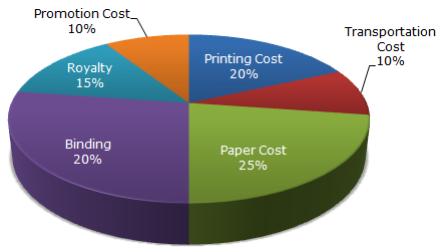# Data Interpretation Questions

03 September 2023

## What is Data Interpretation?

Data Interpretation is the process of making sense out of a collection of data that has been processed. This collection may be present in various forms like bar graphs, line charts and tabular forms and other similar forms.

## Formula's for Data Interpretation

Data Sufficiency problem consists of a question followed by two statements, labeled as Statement (1) and Statement (2), in which certain data are given. You have to decide whether the data given in the statements are sufficient for answering the question. Using the data given in the statements upheld your knowledge of mathematics and everyday facts (such as the number of days in July or the meaning of counterclockwise), you must indicate from
the following answer options that whether:

1. Statement (1) ALONE is sufficient, but statement (2) alone is not sufficient to answer the question asked;

2. Statement (2) ALONE is sufficient, but statement (1) alone is not sufficient to answer the question asked;

3. Both statements (1) and (2) TOGETHER are sufficient to answer the question asked; but NEITHER statement ALONE is sufficient.

5. Statements (1) and (2) TOGETHER are NOT sufficient to answer the question asked,  and additional data specific to the problem are needed.

A statement is sufficient when it guarantees exactly one answer to that question.

## Tips To Solve Data Interpretation Problems:

• Never try to reach final answer as it is not asked. You need to find whether the information  provided is enough to solve the given problem or not.
• Never make any assumption. Use only universal rules { eg. a + b = a + b - (a U b) }
• Try to solve questions by using above strategies
• Solve question step by step. First try to find answer using first statement then second and finally with both then mark the answer
• Even if you find answer with only one statement, then try to find answer with remaining statement as sometimes there is an option that answer can be find with both statements separately.
• Move on quickly and mark answer can’t be found in case you are unable to reach any conclusion with information provided.

The Data interpretation Questions do not require the exam aspirant to find the exact answer. Infact the only thing that they require is to determine whether the statements provided in the question contain enough information for answering it.

## Data Interpretation Questions With Solutions

### Q1)The bar graph given below shows the sales of books (in thousand number) from six branches of a publishing company during two consecutive years 2000 and 2001.

Sales of Books (in thousand numbers) from Six Branches - B1, B2, B3, B4, B5 and B6 of a publishing Company in 2000 and 2001.A) 2:3

B) 3:5

C) 4:5

D) 7:9

A) 68.54%

B) 71.11%

C) 73.17%

D) 75.55%

A) 75%

B) 77.5%

C) 82.5%

D) 87.5%

A) 73

B) 80

C) 83

D) 88

A) 250

B) 310

C) 435

D) 560

### Q6)The following pie-chart shows the percentage distribution of the expenditure incurred in publishing a book. Study the pie-chart and the answer the questions based on it.#### 6.1) If for a certain quantity of books, the publisher has to pay Rs. 30,600 as printing cost, then what will be amount of royalty to be paid for these books?

A) Rs. 19,450

B) Rs. 21,200

C) Rs. 22,950

D) Rs. 26,150

##### Solution :
Let the amount of Royalty to be paid for these books be Rs. r.
Then, 20 : 15 = 30600 : r
=>  r = Rs. (30600 x 15 / 20 ) = Rs. 22,950.

#### 6.2) What is the central angle of the sector corresponding to the expenditure incurred on Royalty?

A) 15°

B) 24°

C) 54°

D) 48°

##### Solution :
Central angle corresponding to Royalty = (15% of 360)°
= (15/100 x 360)°
=54°

#### 6.3)  The price of the book is marked 20% above the C.P. If the marked price of the book is Rs. 180, then what is the cost of the paper used in a single copy of the book?

A) Rs. 36

B) Rs. 37.50

C) Rs. 42

D) Rs. 44.25

##### Solution :
Clearly, marked price of the book = 120% of C.P.
Also, cost of paper = 25% of C.P
Let the cost of paper for a single book be Rs. n.
Then, 120 : 25 = 180 : n => n = Rs. 25 x 180 / 120 = Rs. 37.50 .

#### 6.4) If 5500 copies are published and the transportation cost on them amounts to Rs. 82500, then what should be the selling price of the book so that the publisher can earn a profit of 25%?

A) Rs. 187.50

B) Rs. 191.50

C) Rs. 175

D) Rs. 180

##### Solution :
For the publisher to earn a profit of 25%, S.P. = 125% of C.P.
Also Transportation Cost = 10% of C.P.
Let the S.P. of 5500 books be Rs. x.
Then, 10 : 125 = 82500 : x => x = Rs. 125 x 82500 /10 = Rs. 1031250.
S.P. of one book = Rs. 1031250/5500  = Rs. 187.50 .

#### 6.5) Royalty on the book is less than the printing cost by:

A) 5%

B) 6%

C) 20%

D) 25%

##### Solution :
Printing Cost of book = 20% of C.P.
Royalty on book = 15% of C.P.
Difference = (20% of C.P.) - (15% of C.P) = 5% of C.P.
Percentage difference = (Difference / Printing Cost  x 100 )%
= (5% of C.P. / Printing Cost  x 100) % = 25%.